Courses

# RD Sharma Solutions Ex-8.2, Lines And Angles, Class 9, Maths Class 9 Notes | EduRev

## Class 9 : RD Sharma Solutions Ex-8.2, Lines And Angles, Class 9, Maths Class 9 Notes | EduRev

The document RD Sharma Solutions Ex-8.2, Lines And Angles, Class 9, Maths Class 9 Notes | EduRev is a part of the Class 9 Course RD Sharma Solutions for Class 9 Mathematics.
All you need of Class 9 at this link: Class 9

Q 1: In the below Fig. OA and OB are opposite rays:

(i) If x = 25, what is the value of y?
(ii) If y = 35, what is the value of x?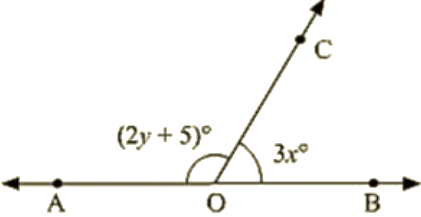Ans :

(i) Given that,

x = 25

Since ∠AOCand∠BOC form a linear pair

∠AOC+∠BOC = 180

Given that ∠AOC = 2y+5 and ∠BOC = 3x

∠AOC+∠BOC = 180

(2y + 5) + 3x = 180

(2y +5) + 3 (25) = 180

2y + 5 + 75 = 180

2y + 80 = 180

2y = 180 – 80  = 100

y = 100/2 = 50

y = 50

(ii) Given that,

y = 35

∠AOC+∠BOC = 180

(2y+5)+3x  = 180

(2(35) + 5) + 3x = 180

(70+5) + 3x = 180

3x = 180 – 75

3x  = 105

x = 35

Q 2 : In the below figure, write all pairs of adjacent angles and all the linear pairs.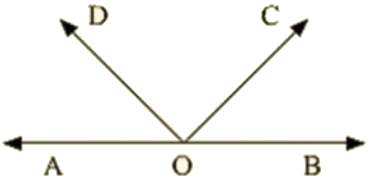Ans : Adjacent angles are :

(i)∠AOC,∠COB

(ii)∠AOD∠BOD

(iii)∠AOD,∠COD

(iv)∠BOC,∠COD

Linear pairs : ∠AOD,∠BOD,∠AOC,∠BOC

Q 3 : In the given below figure, find x. Further find ∠COD,∠AOD,∠BOC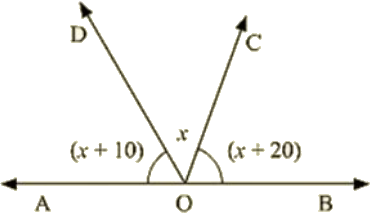Ans : Since  ∠AOD and ∠BOD form a line pair,

∠AOD+∠BOD = 180

∠AOD+∠BOC+∠COD = 180

Given that,

∠AOD = (x+10)∘,∠COD = x,∠BOC = (x+20)

• (x + 10) + x + (x + 20) = 180
• 3x + 30 = 180
• 3x = 180 – 30
• 3x = 150/3
• x = 50

Therefore, ∠AOD=(x+10)

= 50 + 10 = 60

∠COD = x = 50

∠COD = (x+20)

= 50 + 20 = 70

∠AOD = 60∠COD = 50 ∠BOC = 70

Q 4 : In the Given below figure  rays OA, OB, OC, OP and OE have the common end point 0. Show that ∠AOB+∠BOC+∠COD+∠DOE+∠EOA = 360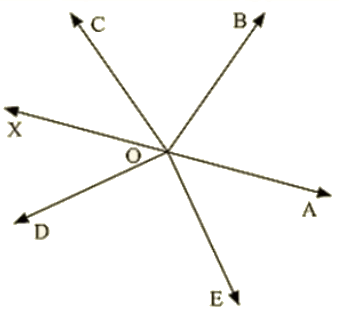Ans : Given that OA,OB,OD and OE have the common end point O.

A ray opposite to OA is drawn

Since ∠AOB,∠BOF are linear pairs,

∠AOB+∠BOF = 180

∠AOB+∠BOC+∠COF = 180  —–(1)

Also,

∠AOEand∠EOF are linear pairs

∠AOE+∠EOF = 180

∠AOE+∠DOF+∠DOE = 180      —–(2)

By adding (1) and (2) equations we get

∠AOB+∠BOC+∠COF+∠AOE+∠DOF+∠DOE = 180

∠AOB+∠BOC+∠COD+∠DOE+∠EOA = 180

Hence proved.

Q 5 : In the Below figure, ∠AOC and ∠BOC form a linear pair. If a – 2b =30, find a and b ?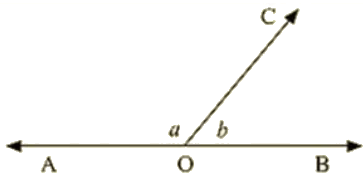Ans : Given that,

∠AOC and ∠BOC form a linear pair

If a – b = 30

∠AOC = a,∠BOC = b

Therefore,  a +b  =180   —–(1)

Given a – 2b = 30       —–(2)

By subtracting (1) and (2)

a+b-a+2b=180-30

• 3b = 150
• b = 150/3
• b = 50

Since a – 2b = 30

a – 2(50) = 30

a = 30 + 100

a = 130

Hence, the values of a and b are 130∘and50∘ respectively.

Q 6 : How many pairs of adjacent angles are formed when two lines intersect at a point ?

Ans : Four pairs of adjacent angles will be formed when two lines intersect at a point.

Considering two lines AB and CD intersecting at O

The 4 pairs are :

(∠AOD,∠DOB),(∠DOB,∠BOC),(∠COA,∠AOD)and(∠BOC,∠COA)

Hence, 4 pairs of adjacent angles are formed when two lines intersect at a point.

Q 7 : How many pairs of adjacent angles , in all, can you name in the figure below ?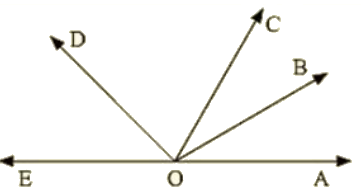Ans : Pairs of adjacent angles are :

∠EOC,∠DOC

∠EOD,∠DOB

∠DOC,∠COB

∠EOD,∠DOA

∠DOC,∠COA

∠BOC,∠BOA

∠BOA,∠BOD

∠BOA,∠BOE

∠EOC,∠COA

∠EOC,∠COB

Hence,  10 pair of adjacent angles.

Q 8 : In the below figure, find value of x ?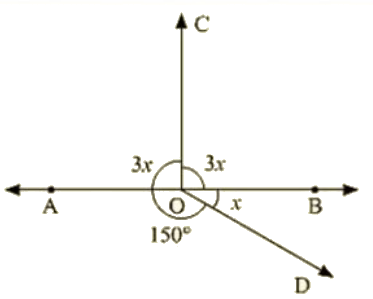Ans : Since the sum of all the angles round a point is equal to 360

• 3x + 3x + 150 + x = 360
• 7x = 360 – 150
• 7x = 210
• x = 210/7
• x = 30

Value of x is 30∘

Q 9 : In the below figure, AOC is a line, find x.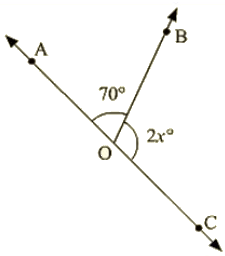Ans : Since ∠AOBand∠BOC are linear pairs,

∠AOB+∠BOC = 180

• 70 + 2x = 180
• 2x = 180 – 70
• 2x = 110
• x = 110/2
• x = 55

Hence, the value of x is 55

Q 10 : In the below figure, POS is a line, Find x ?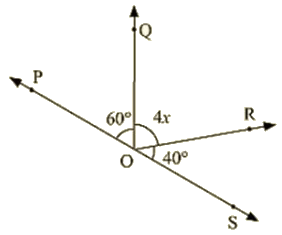Ans : Since ∠POQand∠QOS are linear pairs

∠POQ+∠QOS = 180

• ∠POQ+∠QOR+∠SOR = 180
• 60 + 4x +40 = 180
• 4x = 180 -100
• 4x = 80
• x = 20

Hence, Value of x = 20

Q 11: In the below figure, ACB is a line such that ∠DCA=5xand∠DCB=4x. Find the value of x ?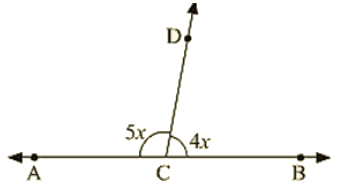Ans: Here, ∠ACD+∠BCD = 180

[Since they are linear pairs]

∠DCA = 5xand∠DCB = 4x

• 5x + 4x = 180
• 9x = 180
• x = 20

Hence, the value of x is 20

Q 12 : In the given figure,  Given ∠POR = 3x and ∠QOR = 2x+10, Find the value of x for which POQ will be a line ?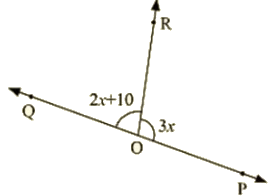Ans : For the case that POR is a line

∠POR and ∠QORarelinearparts

∠POR+∠QOR = 180

Also, given that,

∠POR = 3x and ∠QOR = 2x+10

• 2x + 10 + 3x = 180
• 5x + 10 = 180
• 5x = 180 – 10
• 5x = 170
• x = 34

Hence the value of x is 34

Q 13 : In Fig : a is greater than b by one third of a right angle. Find the value of a and b ?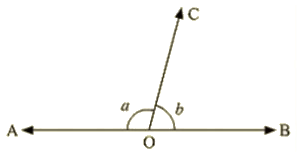Ans : Since a and b are linear

• a + b = 180
• a = 180 – b  —–(1)

From given data, a is greater than b by one third of a right angle

• a = b + 90/3
• a = b + 30
• a – b = 30 —–(2)

Equating (1) and (2)

180 – b = b + 30

• 180 – 30 = 2b
• b = 150 / 2
• b = 75

From (1)

a = 180 – b

• a = 180 – 75
• a = 105

Hence the values of a and b are 105 and 75 respectively.

Q 14 :What value of y would make AOB a line in the below figure, If ∠AOB=4yand∠BOC = (6y+30)?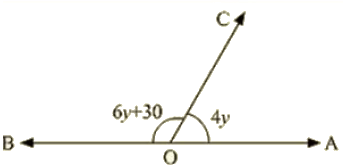Ans :      Since, ∠AOCand∠BOC are linear pairs

∠AOC+∠BOC = 180

• 6y + 30 + 4y = 180
• 10y + 30 = 180
• 10y = 180 – 30
• 10y = 150
• y = 150/10
• y = 15

Hence value of y that will make AOB a line is 15

Q 15 : If the figure below forms a linear pair,

∠EOB=∠FOC=90 and ∠DOC=∠FOG=∠AOB=30

• Find the measure of ∠FOE,∠COBand∠DOE
• Name all the right angles
• Name three pairs of adjacent complementary angles
• Name three pairs of adjacent supplementary angles
• Name three pairs of adjacent angles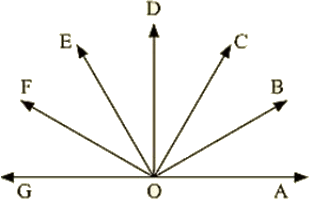Ans :(i) ∠FOE = x,∠DOE = y and ∠BOC = z

Since ∠AOF,∠FOG is a linear pair

• ∠AOF + 30 = 180
• ∠AOF = 180 – 30
• ∠AOF = 150
• ∠AOB+∠BOC+∠COD+∠DOE+∠EOF = 150
• 30 + z + 30 + y + x = 150
• x + y + z =150 – 30 – 30
• x + y + z =90 —–(1)

∠FOC = 90

• ∠FOE+∠EOD+∠DOC = 90
• x + y +30 = 90
• x + y = 90 – 30
• x + y =60 —–(2)

Substituting (2) in (1)

x + y + z = 90

• 60 + z = 90
• z = 90 – 60 = 30

Given BOE = 90

• ∠BOC+∠COD+∠DOE = 90
• 30 + 30 +DOE = 90
• DOE = 90 – 60 = 30
• DOE = x = 30

We also know that,

x + y = 60

• y = 60 – x
• y = 60 – 30
• y = 30

Thus we have ∠FOE = 30,∠COB = 30 and ∠DOE = 30

(ii) Right angles are ∠DOG,∠COF,∠BOF,∠AOD

(iii) Adjacent complementary angles are (∠AOB,∠BOD);(∠AOC,∠COD);(∠BOC,∠COE);

(iv) Adjacent supplementary angles are (∠AOB,∠BOG);(∠AOC,∠COG);(∠AOD,∠DOG);

Q16: In below fig. OP, 0Q, OR and OS are four rays. Prove that: ∠POQ+∠QOR+∠SOR+∠POS=360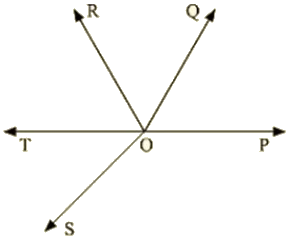Ans:   Given that

OP, OQ, OR and OS are four rays

You need to produce any of the ray OP, OQ, OR and OS backwards to a point in the figure.

Let us produce ray OQ backwards to a point T

So that TOQ is a line

Ray OP stands on the TOQ

Since ∠TOP,∠POQ is a linear pair

∠TOP+∠POQ = 180                          —–(1)

Similarly,

Ray OS stands on the line TOQ

∠TOS+∠SOQ = 180                        —–(2)

But ∠SOQ = ∠SOR+∠QOR                            —–(3)

So, eqn (2) becomes

∠TOS+∠SOR+∠OQR = 180°

Now, adding (1) and (3) you get ∠TOP+∠POQ+∠TOS+∠SOR+∠QOR = 360  —–(4)

∠TOP+∠TOS=∠POS

Eqn: (4)becomes

∠POQ+∠QOR+∠SOR+∠POS = 360

Q 17 : In below fig, ray OS stand on a line POQ. Ray OR and ray OT are angle bisectors of ∠POSand∠SOQrespectively. If ∠POS = x, find ∠ROT ?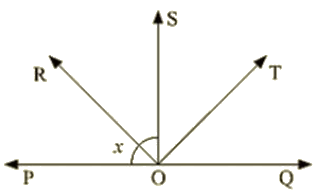Ans : Given,

Ray OS stand on a line POQ

Ray OR and Ray OT are angle bisectors of  ∠POSand∠SOQ respectively

∠POS = x

∠POSand∠SOQ is linear pair

∠POS+∠QOS = 180

x + QOS = 180

• QOS = 180 – x

Now, ray or bisector POS

∠ROS=1/2∠POS

• x/2

ROS = x/2            [Since POS = x ]

Similarly ray OT bisector QOS

∠TOS = 1/2∠QOS

= (180 – x)/2       [ QOS = 180 – x ]

= 90 – x/2

Hence, ∠ROT=∠ROS+∠ROT

= x/2 + 90 – x/2

= 90

∠ROT = 180

Q 18 :  In the below fig, lines PQ and RS intersect each other at point O. If ∠POR:∠ROQ=5:7 . Find all the angles.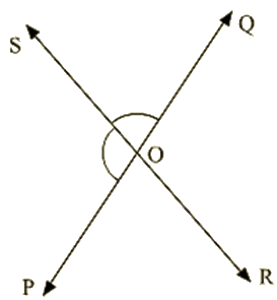Ans : Given

∠POR and ∠ROP is linear pair

∠POR+∠ROP = 180

Given that

∠POR:∠ROQ=5:7

Hence, POR =(5/12)x180=75

Similarly ROQ=(7/7+5) 180 = 105

Now POS = ROQ = 105° [Vertically opposite angles]

Also, SOQ = POR = 75°    [Vertically opposite angles]

Q 19 : In the below fig. POQ is a line. Ray OR is perpendicular to line PQ. OS is another ray lying between rays OP and OR. Prove that ∠ROS = 1/2(∠QOS–∠POS).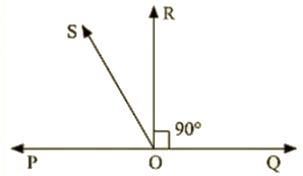Ans : Given that

OR perpendicular

∴ ∠POR=90

∠POS+∠SOR=90         [∵ ∠POR=∠POS+∠SOR]

∠ROS=90°−∠POS         —–(1)

∠QOR = 90 (∵ OR ⊥ PQ)

∠QOS–∠ROS = 90

∠ROS=∠QOS−90

By adding (1) and (2) equations, we get

2∠ROS =∠QOS–∠POS

∠ROS = 1/2(∠QOS–∠POS)

Offer running on EduRev: Apply code STAYHOME200 to get INR 200 off on our premium plan EduRev Infinity!

91 docs

,

,

,

,

,

,

,

,

,

,

,

,

,

,

,

,

,

,

,

,

,

,

,

,

,

,

,

,

,

,

;